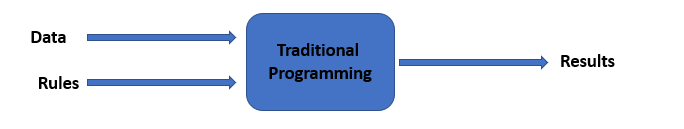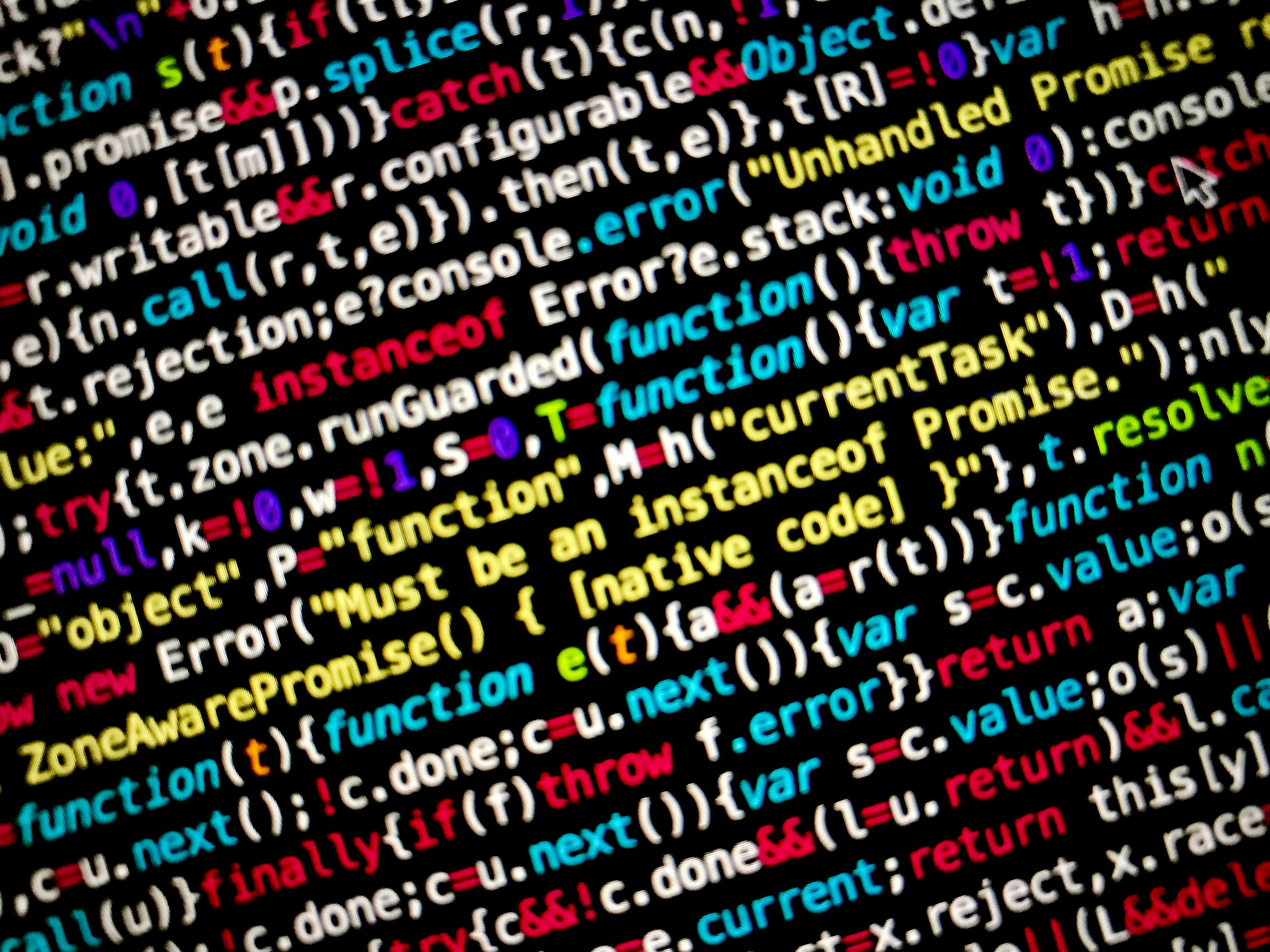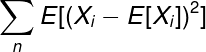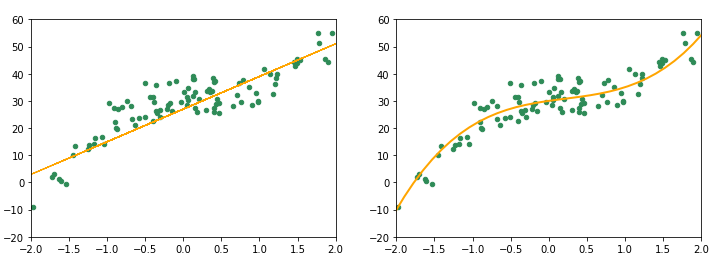1594371180

# The Bias-Variance Tradeoff Should be Considered For Every Model

Questions about the bias-variance tradeoff are used very frequently in interviews for data scientist positions. They often serve to delineate a data scientist that is seasoned and knows their stuff versus one that is junior… and more specifically, as one who is unfamiliar with their options for mitigating prediction error within a model.

# So what is it again?

So bias-variance tradeoff… ever heard of it? If not you’ll want to tune in.

The bias-variance tradeoff is a simple idea, but one that should inform many of the statistical analysis & modeling that you do, primarily when it comes to eliminating error from predictions.

# Where error comes into play

When you create a model, your model will have some error. Makes sense! Nothing new here; what is new is the idea that said error is actually made up of two things… You guessed it, bias & variance! Sorry to drill this in so hard, but this reason that matters is that once you understand the component pieces of your error, then you can determine a plan to minimize it.

There are different methods and approaches you can take to manage and minimize bias or variance, but the act of doing so comes with its considerations. Hence, why it is so pivotal for you as a data scientist to understand the effects of either.

# Let’s break down bias

Bias represents the difference between our prediction and actuals.

# High bias vs low bias

A model that with high bias is one that would garner little from data to then generate predictions. A common phrase you might hear is that a high bias model is ‘over generalized’. It depends very little on the training data to determine its predictions, thus when it comes to generating accurate predictions on your test data… it performs very poorly.

There may be assumptions implicit within our approach that leads to a lack of attention given to those features that would allow a model to generate predictions with greater performance.

Conversely, low bias represents a model that is highly accurate. Thus, it’s something we’d clearly want to minimize.

# What does variance mean for your model?

Variance is pretty much what it sounds like; variance has to do with the distribution of our predictions and how ‘variable’ they are. If you’ve ever heard the term ‘overfitting’; this is effectively an explanation of the outcomes of a high variance model.

#data-science #machine-learning #statistics #data analysis

## Buddha Community1603004400

In the process of building a Predictive Machine learning model, we come across the Bias and Variance errors. The Bias-Variance Tradeoff is one of the most popular tradeoffs in Machine Learning. Here, we will go over what Bias error and Variance error are, sources of these errors and how you can work to reduce these errors in your model.

How does Machine Learning differ from traditional programming?

The high school definition of a program was simple. A program is a set of rules that tells the computer what to do and how to do it. This is one of the main difference between traditional programming and Machine Learning.

In traditional programming, the programmer defines the rules. The rules are usually well defined and a programmer often has to spend a good amount of time debugging code to ensure that the code runs smoothly.In Machine Learning, while we still we still code, we do not define the rules. We build a model and feed it our expected results (supervised ML) or we allow the model come up with its own results (unsupervised ML). The main focus in Machine Learning is to improve the accuracy of the initial guess the model makes.1594560000

## Bias Variance decomposition

We often wonder how to select a method from a pool of machine learning methods which gives best results for a given dataset.

“The process of selecting the best model with appropriate complexity for a specific problem statement is known as model selection”

This brings us to a very important property of statistical learning methods known as bias variance trade off, that emphasizes on how well a model is learning the associations in the training datasets.

In this article, we will discuss what is bias and variance, and how to reduce them. Finally, we will implement a few practical illustrations to see how these concepts can be applied in model building.

So, let’s get started.

There are 3 types of error in predictions due to deviation from the actual truth:

1. Irreducible error
2. Bias
3. Variance

Irreducible error is a measure of noise inherent in the data. There might always be some predictors which have some small effect on the target variable and are not part of our model.

Thus, no matter how good a model we might select, it will not be able to approximate the actual function perfectly, hence leaving the error which cannot be reduced.

So, let’s focus on what we can control while building model i.e. bias and variance and how.

For this purpose, we will use ‘mlxtend’ library (developed by Sebastian Raschka) to calculate ‘bias_variance_decomp’ .1598278920

Supervised Learning can be best understood by the help of Bias-Variance trade-off. The main aim of any model comes under Supervised learning is to estimate the target functions to predict the output with the help of input variables. Supervised learning consists of the Machine learning Algorithms, that are used for the data for its analysis by looking at its previous outcomes. Every action, has its outcomes or final target which helps it to be useful. Supervised Learning takes the help of the actions and its previous outcomes to analyze it and predict the possible outcomes of future. In Supervised Learning every algorithms function on some previous known data which is labeled; labeled here means that every information about the data is given. Algorithms is being trained on that labelled data repeatedly and then machine performs the actions based on that training to predict the outcomes. These predicted outcomes are more or less very similar to the past outcomes. This helps us to take decisions for the actions that hasn’t been occurred yet. Whether it is weather forecasting, predicting stock market price, house/property price, detecting email spam, recommendation system, self-driving car, churn modelling, sale of products etc., Supervised Learning comes into actions. In Supervised Learning, you supervise the learning process, meaning the data that you have collected here is labelled and so you know what input needs to be mapped to what output. it is the process of making an algorithm to learn to map an input to a particular output. This is achieved using the labelled datasets that you have collected. If the mapping is correct, the algorithm has successfully learned. Else, you make the necessary changes to the algorithm so that it can learn correctly. Supervised Learning algorithms can help make predictions for new unseen data that we obtain later in the future. It is as same as the teacher-student scenario. A teacher teaches the students to learn from the book (labelled datasets), and students learn from it and later on gives the test (prediction of algorithm) to pass. If the student fails (overfitting or underfitting), teacher tune the students (hyperparameter tuning) to perform better later on. But theirs a lot to catch-up between what is an ideal condition and what in practical possible. As no students (Algorithms) or teacher (datasets) can be 100 percent true or correct in their work. Same way, there are many advantages and disadvantages of every model and data that is been feeded into the model. Datasets might be unbalanced, consists of many missing values, improperly shaped and sized, can contains many outliers that makes any model task difficult to perform. Similarly, every model has its disadvantages or makes error in mapping the outputs. I will talk about these errors that prevent models to perform best and how can we overcome those errors.Photo by Max Chen on Unsplash

Before proceeding with the model training, we should know about the errors (bias and variance) related to it. If we know about it, not only it would help us with better model training but also, helps us to deal with underfitting and overfitting of model.

This predictive error is of three types:

1. Bias

2. Variance

3. Irreducible error1594330800

## How to measure the variance of a statistical model

This article assumes that you understand and know how to build regression or classification models.

The error of any statistical model is composed of three parts — bias, variance and noise. In layman’s terms, bias is the inverse of the accuracy of predictions. And variance refers to the degree to which the predictions are spread out. Noise, on the other hand, is random fluctuation that cannot be expressed systematically.

However, the above definitions are vague and we need to inspect them from a mathematical perspective. In this article, I will stress on variance — the more perplexing demon that haunts our models.

# A short note on Variance

When we allow our models the flexibility to uselessly learn complex relationships over the training data, it loses the ability to generalize. Most of the times, this flexibility is provided through features i.e when the data has a large number of features (sometimes more than the number of observations). It could also be due to a complex neural network architecture or an excessively small training dataset.

What results from this is a model which also learns the noise in the training data; consequently, when we try to make predictions on unseen data, the model misfires.

_Variance is also responsible for the differences in predictions on the same observation in different “realizations” of the model. _We will use this point later to find an exact value for the variance.

# Mathematical explanation

Let Xᵢ be the population of predictions made by model M on observation i. If we take a sample of size n values, the variance will be:Now that was something we already knew. However, we need to calculate the variance of the whole population (which is equivalent to the variance of the statistical model that generated it) and we are not quite there yet. There is one concept we need to understand before that — bootstrap resampling.

Note that this formula of variance assumes that the outcome of the model is a continuous variable — this happens in regression. In the case of classification, the outcome is 0/1 and thus, we would have to measure the variance differently. You can find the explanation to that in this paper.

## Bootstrap Resampling

Often we don’t have access to an entire population to be able to calculate a statistic such as variance or mean. In such cases, we make use of bootstrap sub-samples.

The principle of bootstrapping suggests that if we take a large number of sub-samples of size n with replacement from a sample of size n, then it is an approximation of taking those samples from the original population.

We find the sample statistic on each of these sub-samples and take their mean to estimate the statistic with respect to the population. The number of sub-samples we take is only limited by time and space constraints; however, the more you take, the more accurate will be your result.

## Realizations of a model

Let _M _be our statistical model. A realization of M is a mapping from input to output. When we train M on a particular input, we obtain a specific realization of the model. We can obtain more realizations by training the model on sub-samples from the input data.

#machine-learning #bias-variance #variance-analysis #variance #sensitivity-analysis #data analysis1594641600

Some facts just mess up in our minds and then it gets hard to recall what’s what. I had a similar experience with Bias & Variance, in terms of recalling the difference between the two. And the fact that you are here suggests that you too are muddled by the terms.

So let’s understand what Bias and Variance are, what Bias-Variance Trade-off is, and how they play an inevitable role in Machine Learning.

## The Bias

Let me ask you a question. Why do humans get biased when they do? Or what motivates them to show some bias every now and then?

I’m sure you had a good answer or many good answers. But to summarise them all, the most fundamental reason that we see bias around us is —_ ease of mind._

Being humans, it’s easy to incline our thoughts and favors towards something we like, we admire, or something we think is right, without bending our thoughts much.

For most of our life’s decisions, we don’t want to put our brains into analyzing each and every scenario. Now one might be investigative, meticulous, or quite systematic while doing things that are important and consequential, but for the most part, we are too lazy to do that.

But how this human intuition of being biased is related to Machine Learning? Let’s understand how.

Consider the figure below.

One could easily guess that this figure represents Simple Linear Regression, which is an _inflexible _model that assumes a linear relationship between input and output variables. This assumption, approximation, and restriction introduce _bias _to this model.

Hence bias refers to the error which is observed while approximating a complex problem using a simple (or restrictive) model.

This analogy between humans and machines could be a great way to understand that inflexibility brings bias.

Observe the figure below. The plots represent two different models that were used to fit the same data. Which one do you think will result in higher bias?The plot on the right is quite more flexible than the one on the left. It fits more smoothly with the data. On the other hand, the plot on the left represents a poorly fitted model, which assumes a linear relationship in data. This poor-fitting due to high bias is also known as underfitting. Underfitting results in poor performance and low accuracies and can be rectified if needed by using more flexible models.

Let’s summarise the key points about bias:

• Bias is introduced when restrictive (inflexible) models are used to solve complex problems
• As the flexibility of a model increases, the bias starts decreasing for training data.
• Bias can cause underfitting, which further leads to poor performance and predictions.

So how can we get rid of this bias? We can build a more flexible model to fit our data and remove underfitting.

So should we keep building more complex models until we reduce the error to its minimum? Let’s try and do that with some randomly generated data.

#machine-learning #bias #variance #data-science #data analysis